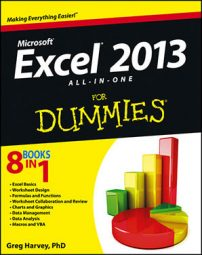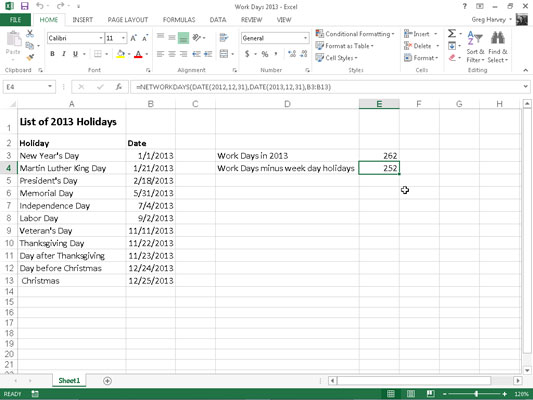##### Excel 2013 All-in-One For DummiesExcel 2013 includes other special Date functions in the Date and Time category in the Insert Function dialog box. These particular Date functions expand your abilities to do date calculations in the worksheet — especially those that work only with normal workdays, Monday through Friday.

## EDATE function

The EDATE (for Elapsed Date) function calculates a future or past date that is so many months ahead or behind the date that you specify as its start_date argument. You can use the EDATE function to quickly determine the particular date at a particular interval in the future or past (for example, three months ahead or one month ago).

The EDATE function takes two arguments:

`EDATE(start_date,months)`

The start_date argument is the date serial number that you want used as the base date. The months argument is a positive (for future dates) or negative (for past dates) integer that represents the number of months ahead or months past to calculate.

For example, suppose that you enter the following EDATE function in a cell:

`=EDATE(DATE(2012,1, 31),1)`

Excel returns the date serial number, 40968, which becomes 2/29/2012 when you apply the first Date format to its cell.

## EOMONTH function

The EOMONTH (for End of Month) function calculates the last day of the month that is so many months ahead or behind the date that you specify as its start_date argument. You can use the EOMONTH function to quickly determine the end of the month at a set interval in the future or past.

For example, suppose that you enter the following EOMONTH function in a cell:

`=EOMONTH(DATE(2013,1,1),1)`

Excel returns the date serial number, 41333, which becomes 2/28/2013 when you apply the first Date format to its cell.

## NETWORKDAYS function

The NETWORKDAYS function returns the number of workdays that exist between a starting date and ending date that you specify as arguments:

`NETWORKDAYS(start_date,end_date,[holidays])`

When using this function, you can also specify a cell range in the worksheet or array constant to use as an optional holidays argument that lists the state, federal, and floating holidays observed by your company. Excel then excludes any dates listed in the holidays argument when they occur in between start_date and end_date arguments.

In this worksheet shown, a list is created in the cell range B3:B13 with all the observed holidays in the calendar year 2013. Then the following NETWORKDAYS function was entered in cell E4:`NETWORKDAYS(DATE(2012,12,31),DATE(2013,12,31),B3:B13)`

The preceding function calculates the number of workdays between December 31, 2012, and December 31, 2013 (262 total work days), and then subtracts the dates listed in the cell range B3:B13 if they fall on a weekday.

As 10 of the 11 holidays in the range B3:B13 happen to fall on a weekday in the year 2013, the number of workdays between December 31, 2012, and December 31, 2013, is calculated as 252 in cell E4 (262–10=252).

## WEEKNUM function

The WEEKNUM function returns a number indicating where the week in a particular date falls within the year. This function takes the following arguments:

`WEEKNUM(serial_number,[return_type])`

In this function, the serial_number argument is the date whose week in the year you want to determine. The optional return_type argument is number 1 or 2, where number 1 (or omitted) indicates that the new week begins on Sunday and weekdays are numbered from 1 to 7. Number 2 indicates that the new week begins on Monday and that weekdays are also numbered from 1 to 7.

For example, if you enter the following WEEKNUM function in a cell:

`=WEEKNUM(DATE(2014,1,19))`

Excel returns the number 4, indicating that the week containing the date January 19, 2014, is the fourth week in the year when the Sunday is considered to be the first day of the week. (January 19, 2014, falls on a Sunday.)

Note that if you had added 2 as the optional return-type argument, Excel would return 3 as the result because January 19, 2014, is deemed to fall on the last day of the third week of the year when Monday is considered the first day of the week.

## WORKDAY function

You can use the WORKDAY function to find out the date that is so many workdays before or after a particular date. This function takes the following arguments:

`WORKDAY(start_date,days,[holidays])`

The start_date argument is the initial date that you want used in calculating the date of the workday that falls so many days before or after it. The days argument is the number of workdays ahead (positive integer) or behind (negative integer) the start_date.

The optional holidays argument is an array constant or cell range that contains the dates of the holidays that should be excluded (when they fall on a weekday) in calculating the new date.

For example, suppose that you want to determine a due date for a report that is 30 workdays after February 1, 2013, by using the same holiday schedule entered in the cell range B3:B13 in the Work Days 2013 workbook. To do this, you enter the following formula:

`=WORKDAY(DATE(2013,2,1),30,B3:B11)`

Excel then returns the serial number 41351 to the cell, which then appears as March 18, 2013 (the day after St. Patrick’s Day), when you format it with the Short Date format.

## YEARFRAC function

The YEARFRAC (for Year Fraction) function enables you to calculate the fraction of the year, which is computed from the number of days between two dates. You can use the YEARFRAC function to determine the proportion of a whole year’s benefits or obligations to assign to a specific period.

The YEARFRAC function uses the following arguments:

`YEARFRAC(start_date,end_date,[basis])`

The optional basis argument in the YEARFRAC function is a number between 0 and 4 that determines the day count basis to use in determining the fractional part of the year:

• 0 (or omitted) to base it on the U.S. (NASD) method of 30/360

• 1 to base the fraction on actual days/actual days

• 2 to base the fraction on actual days/360

• 3 to base the fraction on actual days/365

• 4 to base the fraction on the European method of 30/360

For example, if you enter the following YEARFRAC formula in a cell to find what percentage of the year remains as of October 15, 2013:

`=YEARFRAC(DATE(2013,10,15),DATE(2013,12,31))`

Excel returns the decimal value 0.2111111 to the cell, indicating that just over 21 percent of the year remains.